# ISEE Middle Level Quantitative : Numbers and Operations

## Example Questions

### Example Question #682 : Isee Middle Level (Grades 7 8) Quantitative Reasoning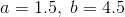Which is the greater quantity?

(A)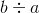(B)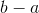(B) is greater

(A) is greater

(A) and (B) are equal

It is impossible to determine which is greater from the information given

(A) and (B) are equal

Explanation: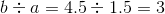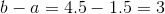The two quantities are equal.

### Example Question #683 : Isee Middle Level (Grades 7 8) Quantitative Reasoning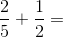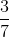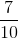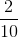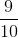Explanation:

When adding fractions with different denominators, you must first find a common denominator. Some multiples of 2 and 5 are:

2: 2, 4, 6, 8, 10...

5: 5, 10, 15, 20...

The first multiple 2 and 5 have in common is 10. Change each fraction accordingly so that the denominator of each is 10.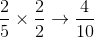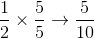The problem now looks like this: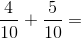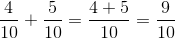### Example Question #684 : Isee Middle Level (Grades 7 8) Quantitative Reasoning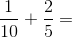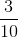Explanation:

When adding fractions with different denominators, first change the fractions so that the denominators are equal. To do this, find the least common multiple of 5 and 10. Some multiples of 5 and 10 are:

5: 5, 10, 15, 20...

10: 10, 20, 30, 40...

Since the first multiple shared by 5 and 10 is 10, change the fractions so that their denominators equal 10.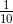already has a denominator of 10, so there is no need to change it.The problem now looks like this: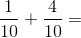Add the fractions by finding the sum of the numerators.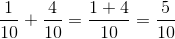When possible, always reduce your fraction. In this case, both 5 and 10 are divisible by 5.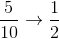### Example Question #685 : Isee Middle Level (Grades 7 8) Quantitative Reasoning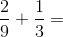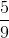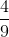Explanation:

When adding fractions with different denominators, first change the fractions so that the denominators are equal. To do this, find the least common multiple of 3 and 9. Some multiples of 3 and 9 are:

3: 3, 6, 9, 12...

9: 9, 18, 27, 36...

Since the first multiple shared by 3 and 9 is 9, change the fractions so that their denominators equal 9.already has a denominator of 9, so there is no need to change it.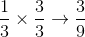The problem now looks like this: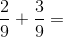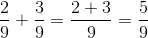### Example Question #686 : Isee Middle Level (Grades 7 8) Quantitative Reasoningis a positive integer. Which is the greater quantity?

(a)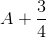(b)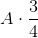(a) and (b) are equal

(a) is the greater quantity

It is impossible to determine which is greater from the information given

(b) is the greater quantity

(a) is the greater quantity

Explanation: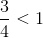andis positive, so by the multiplication property of inequality,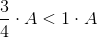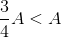Also,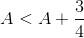,

so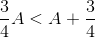### Example Question #687 : Isee Middle Level (Grades 7 8) Quantitative Reasoning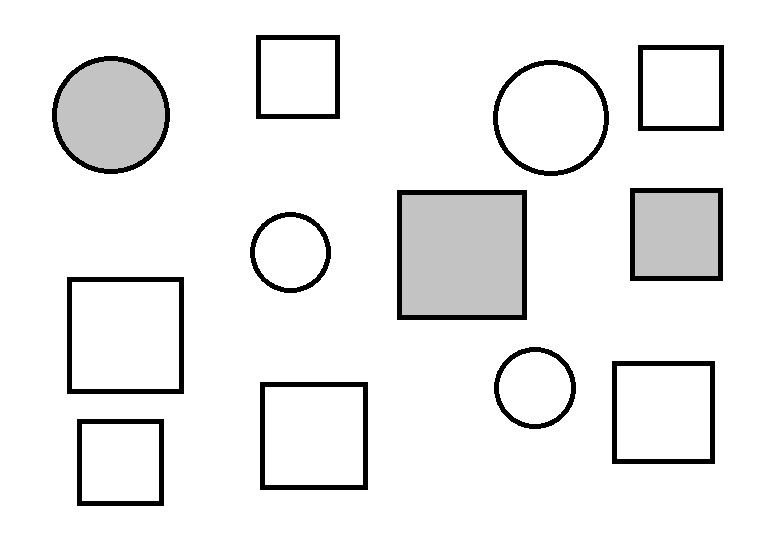Which is the greater quantity?

(a) The fraction of the circles that are shaded

(b) The fraction of the squares that are shaded

It is impossible to determine which is greater from the information given

(a) and (b) are equal

(b) is the greater quantity

(a) is the greater quantity

(a) and (b) are equal

Explanation:

1 of the 4 circles in the diagram -of the circles - are shaded, as are 2 of the 8 squares -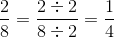of them.

### Example Question #688 : Isee Middle Level (Grades 7 8) Quantitative Reasoning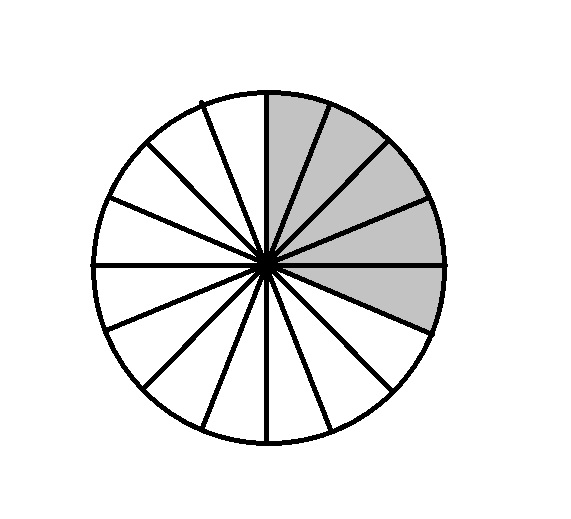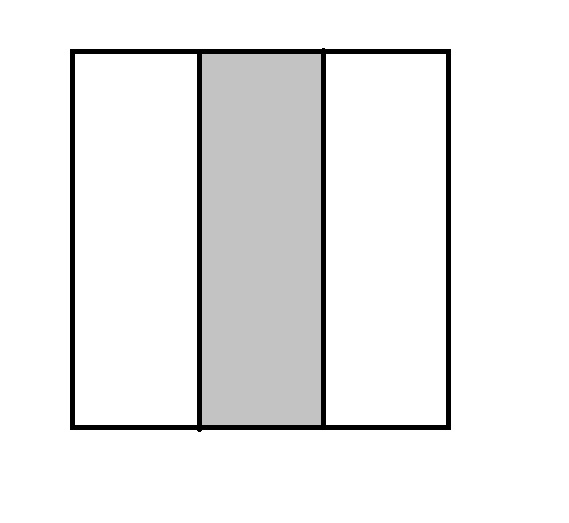Refer to the above figures. The circle and the square are both divided into regions of equal size and shape.

Which is the greater quantity?

(a) The fraction of the circle that is white

(b) The fraction of the square that is white

It is impossible to determine which is greater from the information given

(b) is the greater quantity

(a) is the greater quantity

(a) and (b) are equal

(a) is the greater quantity

Explanation:

The circle is divided into sixteen regions of equal size, eleven of which are white; this is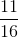of the circle. The square is divided into three regions of equal size, two of which are white; this isof the circle.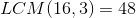, so we can compare the fractions by rewriting them with this denominator: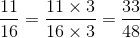and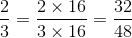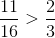, making the fraction of the circle that is white the greater fraction.

### Example Question #689 : Isee Middle Level (Grades 7 8) Quantitative Reasoning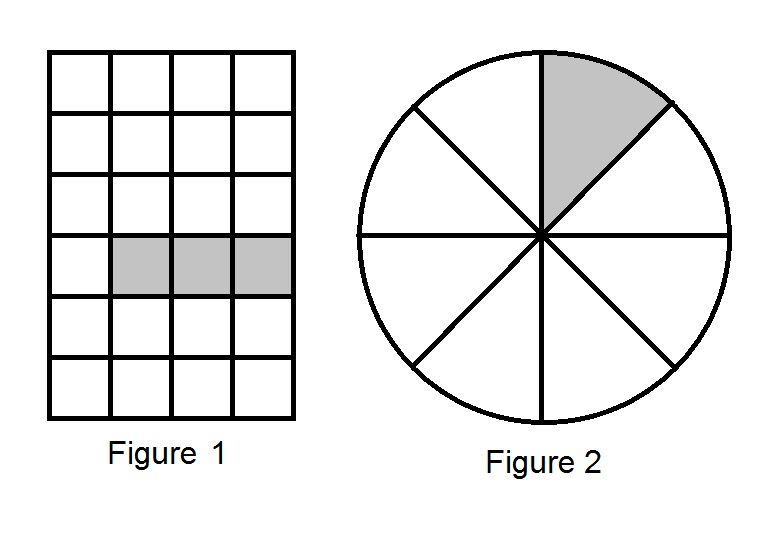Refer to the above diagrams. Each figure is divided into sections of equal size and shape.

Which is the greater quantity?

(a) The fraction of Figure 1 that is shaded

(b) The fraction of Figure 2 that is shaded

(b) is the greater quantity

It is impossible to determine which is greater from the information given

(a) and (b) are equal

(a) is the greater quantity

(a) and (b) are equal

Explanation:

Figure 1 is a rectangle divided into 24 squares of equal size; 3 of the squares are shaded, which means that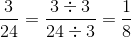of Figure 1 is shaded.

Figure 2 is a circle  divided into 8 sectors of equal size; 1 is shaded, which means thatof Figure 2 is shaded.

The fractions are equal.

### Example Question #690 : Isee Middle Level (Grades 7 8) Quantitative Reasoning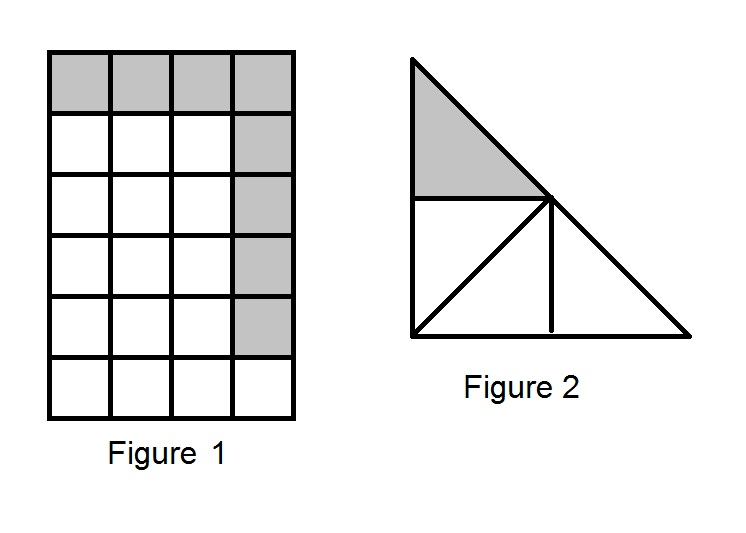Refer to the above diagrams. Each figure is divided into sections of equal size and shape.

Which is the greater quantity?

(a) The fraction of Figure 1 that is shaded

(b) The fraction of Figure 2 that is shaded

(a) is the greater quantity

It is impossible to determine which is greater from the information given

(b) is the greater quantity

(a) and (b) are equal

(a) is the greater quantity

Explanation:

Figure 1 is a rectangle divided into 24 squares of equal size; 8 of the squares are shaded, which means that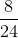of Figure 1 is shaded.

Figure 2 is a triangle divided into 4 triangles of equal size; 1 is shaded, which means that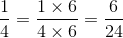of Figure 2 is shaded.

The fraction of Figure 1 that is shaded is the greater quantity.

### Example Question #691 : Isee Middle Level (Grades 7 8) Quantitative Reasoning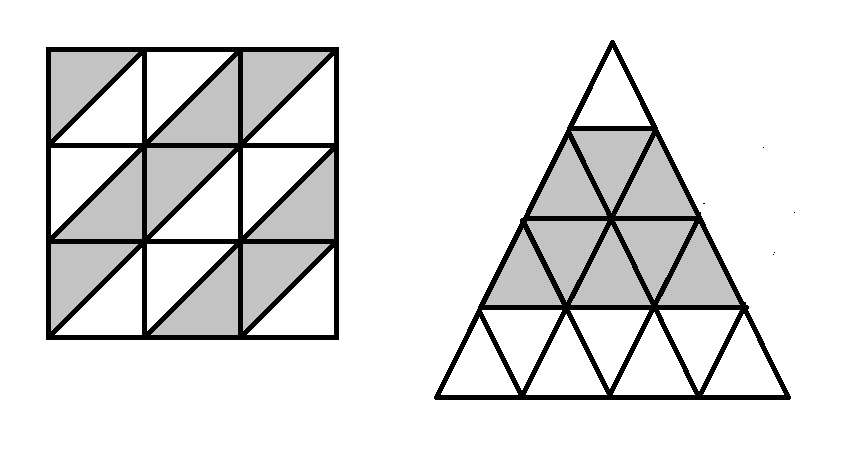The square and the triangle in the above diagram are both equally divided. Which is the greater quantity?

(a) The fraction of the square that is shaded

(b) The fraction of the triangle that is shaded

(a) is the greater quantity

(b) is the greater quantity

It is impossible to determine which is greater from the information given

(a) and (b) are equal

(a) and (b) are equal

Explanation:

The square is divided into eighteen triangles of equal size and shape; nine are shaded, so the fraction of the square that is shaded is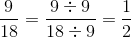The triangle is divided into sixteen triangles of equal size and shape; eight are shaded, so the fraction of the square that is shaded is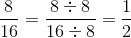.

The fractions are equal.

### All ISEE Middle Level Quantitative Resources